Question

# The enthalpy of fusion of cadmium at its normal melting point of 321 °C is 6.11...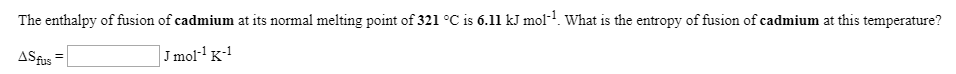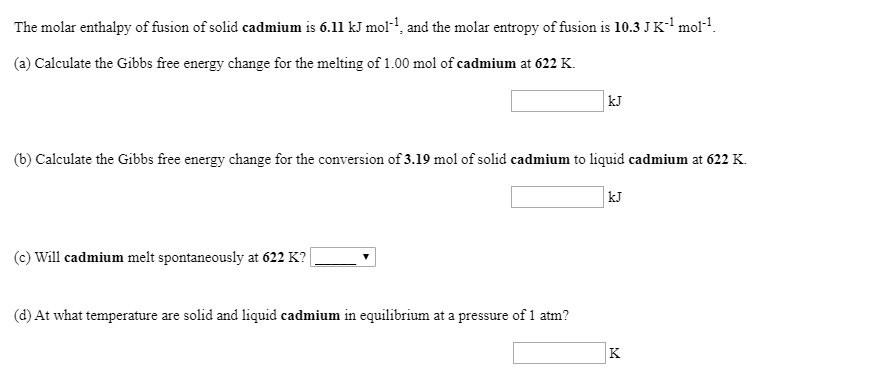The enthalpy of fusion of cadmium at its normal melting point of 321 °C is 6.11 kJ mol? What is the entropy of fusion of cadmium at this temperature? ASfus = J mol-K-1
The molar enthalpy of fusion of solid cadmium is 6.11 kJ mol-1, and the molar entropy of fusion is 10.3 JK+mol-1. (a) Calculate the Gibbs free energy change for the melting of 1.00 mol of cadmium at 622 K. (b) Calculate the Gibbs free energy change for the conversion of 3.19 mol of solid cadmium to liquid cadmium at 622 K. (c) Will cadmium melt spontaneously at 622 K? __ (d) At what temperature are solid and liquid cadmium in equilibrium at a pressure of 1 atm?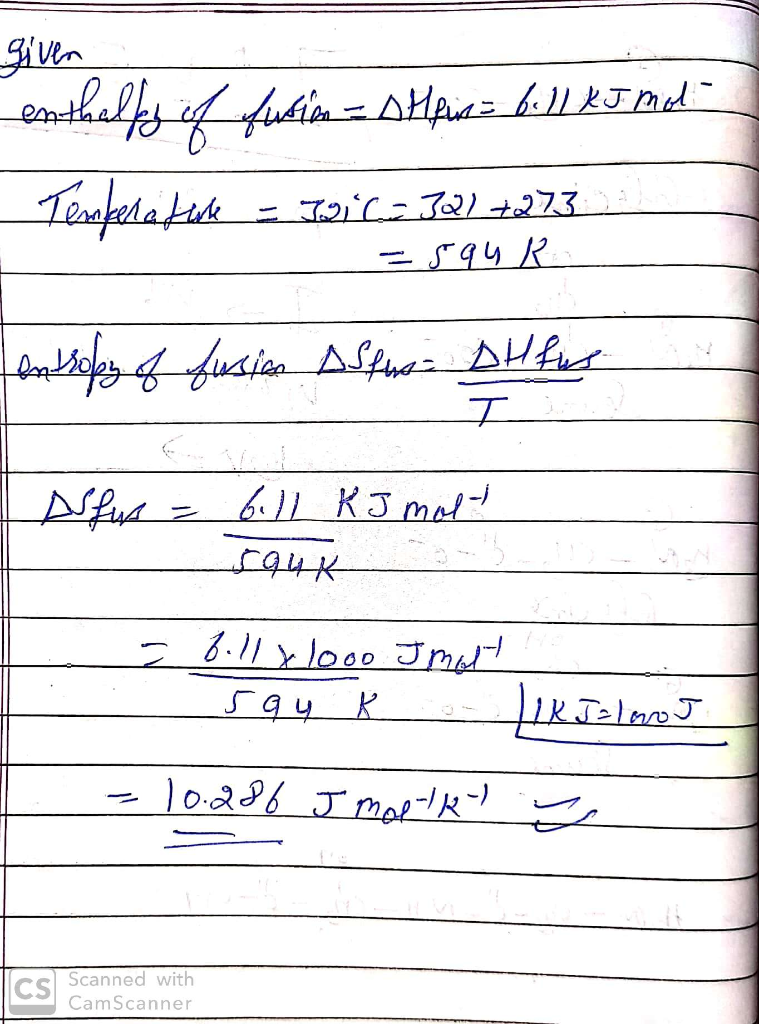#### Earn Coins

Coins can be redeemed for fabulous gifts.

Similar Homework Help Questions
• ### The molar enthalpy of fusion of solid bismuth is 11.0 kJ mol-1, and the molar entropy...

The molar enthalpy of fusion of solid bismuth is 11.0 kJ mol-1, and the molar entropy of fusion is 20.2 J K-1 mol-1. (a) Calculate the Gibbs free energy change for the melting of 1.00 mol of bismuth at 575 K. kJ (b) Calculate the Gibbs free energy change for the conversion of 5.12 mol of solid bismuth to liquid bismuth at 575 K. kJ (c) Will bismuth melt spontaneously at 575 K? (d) At what temperature are solid and...

• ### The enthalpy of fusion of manganese at its normal melting point of 1244 ? is 14.6...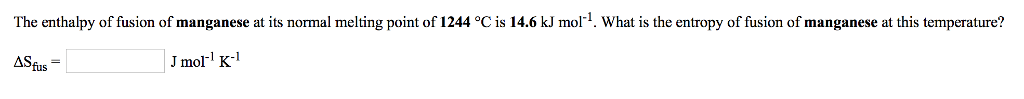The enthalpy of fusion of manganese at its normal melting point of 1244 ? is 14.6 kJ mol-1 What is the entropy of fusion of manganese at this temperature? ASfus Jmol K

• ### The enthalpy of fusion of bismuth at its normal melting point of 271 °C is 11.0...

The enthalpy of fusion of bismuth at its normal melting point of 271 °C is 11.0 kJ mol-1. What is the entropy of fusion of bismuth at this temperature? ΔSfus =  J mol-1 K-1

• ### 14. For bismuth, Bi, the heat of fusion at its normal melting point of 271 °C...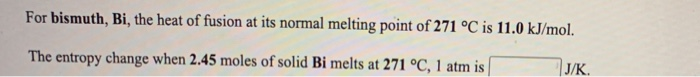14. For bismuth, Bi, the heat of fusion at its normal melting point of 271 °C is 11.0 kJ/mol. The entropy change when 2.45 moles of solid Bi melts at 271 °C, 1 atm is J/K. For magnesium, Mg, the heat of fusion at its normal melting point of 649 °C is 9.0 kJ/mol. The entropy change when 1.72 moles of liquid Mg freezes at 649 °C, 1 atm is J/K

• ### Calculation of enthalpy and entropy of fusion of an unknown solid..?

The molar volume of a certain solid is 142.0 cm3/mol at 1.00 atm and 427.15 K, its melting temperature. The molar volume of the liquid at this temperature and pressure is 152.6 cm3/mol. At 1.2 MPa the melting temperature changes to 429.26 K. Calculate the enthalpy and entropy of fusion of the solid.

• ### Be sure to answer all parts. The molar heats of fusion and vaporization of a certain...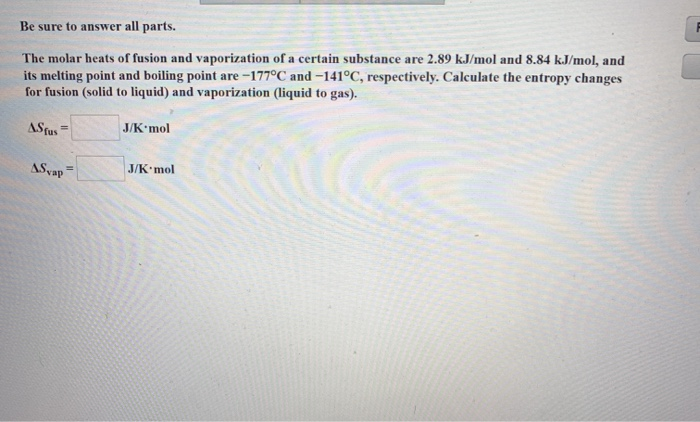Be sure to answer all parts. The molar heats of fusion and vaporization of a certain substance are 2.89 kJ/mol and 8.84 kJ/mol, and its melting point and boiling point are -177°C and -141°C, respectively. Calculate the entropy changes for fusion (solid to liquid) and vaporization (liquid to gas). ASfus J/K mol AS vap = J/K mol

• ### For ethanol, C2H5OH, the heat of fusion at its normal melting point of -115 °C is...

For ethanol, C2H5OH, the heat of fusion at its normal melting point of -115 °C is 5.0 kJ/mol. The entropy change when 1.84 moles of solid C2H5OH melts at -115 °C, 1 atm is J/K.

• ### For copper, Cu, the heat of fusion at its normal melting point of 1083 °C is...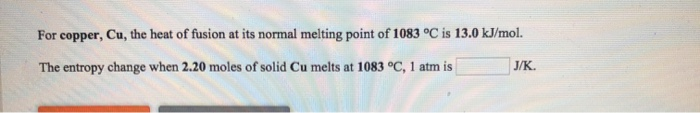For copper, Cu, the heat of fusion at its normal melting point of 1083 °C is 13.0 kJ/mol. The entropy change when 2.20 moles of solid Cu melts at 1083 °C, 1 atm is J/K

• ### The normal melting point of sodium chloride is 801°C, its enthalpy of fusion is 28.8 kJ/mol,...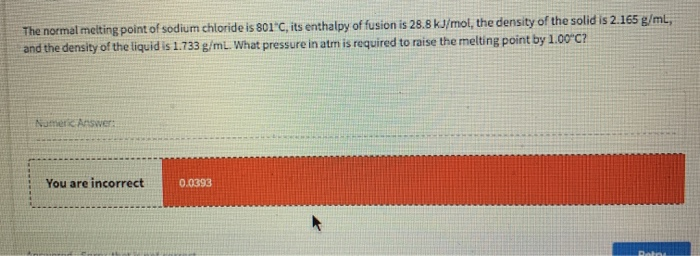The normal melting point of sodium chloride is 801°C, its enthalpy of fusion is 28.8 kJ/mol, the density of the solid is 2.165 g/ml, and the density of the liquid is 1.733 g/ml. What pressure in atm is required to raise the melting point by 1.00°C? Nume Answer: You are incorrect 0.0393

• ### Gallium has a melting point of 29.8oC and an enthalpy of fusion of 5.59 kJ/mol. Calculate...

Gallium has a melting point of 29.8oC and an enthalpy of fusion of 5.59 kJ/mol. Calculate the change in entropy of the system, surroundings, and universe to show that the following process is spontaneous if 10.0 grams of liquid gallium is left in a 20.0oC room.Ga (l, 29.8oC) --> Ga (s, 29.8oC)